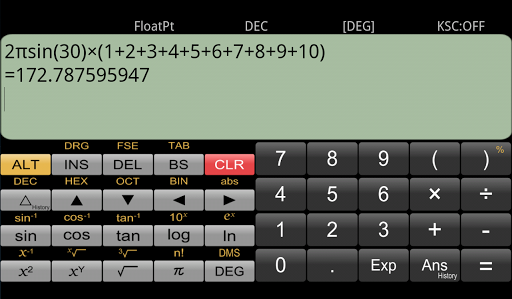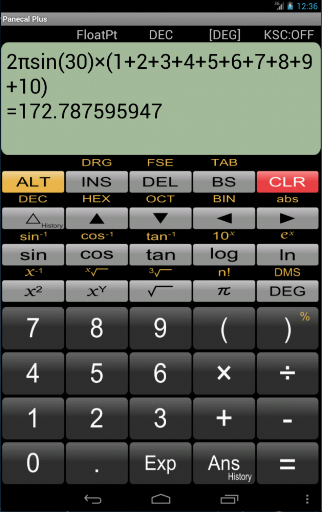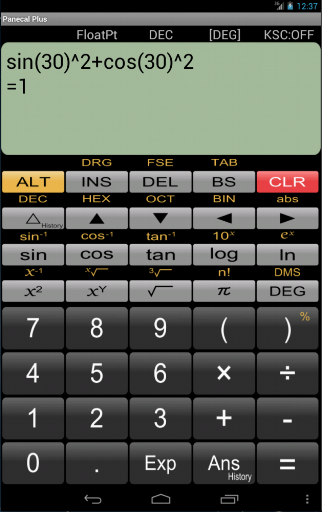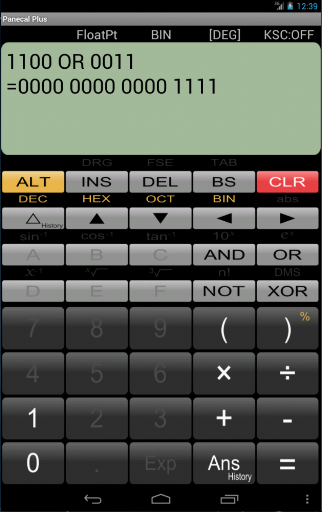More

Scientific calculator with multi-line formula view.
A multiline scientific calculator which can input a numerical formula.

[Features]
* Re-edit the numerical formula by △ key.
* A vibration and orange color key input confirmation. You can modify the vibration length in ALT+Setup.
* Arithmetic, trigonometric, inverse trigonometric, exponential, logarithmic function, power, power root function, factorial, and corresponds to the absolute value operations.
* FloatPt, Fix, Sci, Eng mode.
* BS key, DEL key, INS key.

••••